Courses

# Concentration Cell - Electrochemistry Chemistry Notes | EduRev

## Chemistry : Concentration Cell - Electrochemistry Chemistry Notes | EduRev

The document Concentration Cell - Electrochemistry Chemistry Notes | EduRev is a part of the Chemistry Course Physical Chemistry.
All you need of Chemistry at this link: Chemistry

CONCENTRATION CELL
The cells in which electrical current is produced due to transport of a substance from higher to lower concentration. Concentration gradient may arise either in electrode material or in electrolyte.  Thus there are two types of concentration  cell .
(i) Electrode concentration cell
(ii) Electrolyte concentration cell

Electrode Gas concentration cell

Pt, H2(P1) | H+(C) | H2(P2), Pt
Here, hydrogen gas is bubbled at two different partial pressures at electrode dipped in the solution of same electrolyte.
Cell process :    1 / 2H2 (p1)→H+ (c)+e -       (Anode process)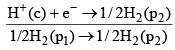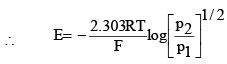or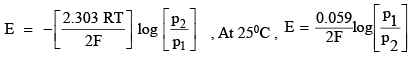For sontaneity of such cell reaction, p1> p2

Electrolyte concentration cells:

Zn(s) | ZnSO4 (C1) || ZnSO4 (C2) | Zn(s)

In such cells, concentration gradient arise in electrolyte solutions. Cell process may be given as,

Zn ( s ) → Zn 2+ ( C1 ) + 2e - (Anodic process)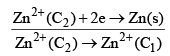(Over all process)
∴  From Nernst equation, we have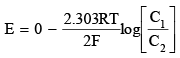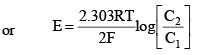For spontanity of such cell reaction, C2> C1

CONCNETRATION CELLS WITHOUT LIQUID JUNCTION POTENTIAL

Concentration cells are made up of two half-cells which are similar chemcially but differ in the activity of some comon component. The common component may be the electrode or the electrolytic solution.
The emf of the cell is due to the differnece of activity of the common component. We descrribe below three categories of concentration cells without liquid junction.

Cells with Amalgam Electrodes

Pb(Hg)(aPb = a1) | Pb2+ (aPb2+) | Pb(Hg) (aPb = a2)

 Electrode                          Reduction reaction                         Reduction Potential Right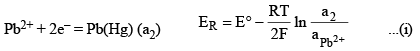Left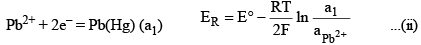Substracting Eq. (ii) from eq. (i), we get

Pb(Hg)(a1) = Pb(Hg)(a2)

and Ecell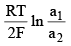Cells with gas electrodes operating at differnet Pressures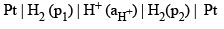We have

 Electrode                    Reduction reaction                           Reduction potential Right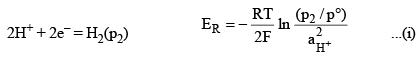Left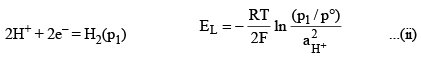Substracting Eq. (ii) from eq. (i), we get

H2(p1) = H2(p2)

and Ecell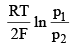Cells with differnet Electrolytic Activities

This type of cells can be formed by making a composite cell out of two cells differing only in the activity of the electrolytic solution. For example, the cell

Pt | H2(p) | H+Cl(a±)1 | AgCl | Ag
may be combined with a cell

Pt | H2(p) | H+Cl(a±)2 | AgCl | Ag
to give the following composite cell.
Pt | H2(p) | H+Cl(a±)1 | AgCl(s) | Ag – Ag | AgCl | H+Cl(a±)2 | H2(p) | Pt

Ecell = EL + ER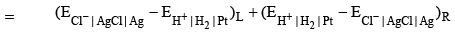Writing the Nernst equation for each potential, we obtain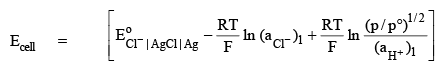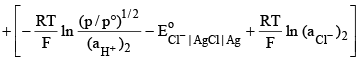or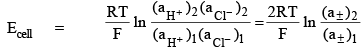The net cell reaction is obtained by adding the individual cell reactions. Thus, we have

 Cell                                   Cell reaction Right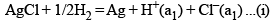Left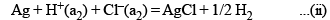Adding eqs. (i) and (ii), we get

H+(a2) + Cl(a2) = H+(a1) + Cl(a1)

Note that the emf o the cell may be derived directly from the cell reaction.
If one faraday of electricity is withdrawn from the cell, the net result that is produced is the transfer of 1 mol of each of hydrogen and chloride ions from the right-side cell to the left-side cell. A cell of this type is called a concentration cell wtihout transference. The operation of cell is not accompanied by the direct transfer of electrolyte from one solution to the other.

CONCENTRATION CELL WITH LIQUID JUNCTION POTENTIAL

Development of Liquid Junction Potential In a cell if two electrolytic solutions of different concentration are in contact with each other, a potential difference develops across the boundary of the two solutions This potential difference is called the liquid junction potential or the diffusion potential. It arises because of the difference in the rates of diffusion of positive and negative ions from more concentrated solution to less concentration solution.
The rate of diffusion of an ion is determined by its transference number. To illustrate how the liquid junction potential arises.

CELL IN WHICH ELECTRODES ARE REVERSIBLE WITH RESPECT TO CATION

A Typical Example
Consider the cell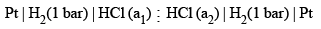Working of the cell

(i)  Electrode reaction at anode 1/2 H2(1 bar) →  H+(a1) + e
(ii)  Electrode reaction at cathode H+(a2) + e- →  1/2H2(1 bar)
(iii)  Transfer of t+ mole of H+ from left to right t+ H+(a1)  →  t+ H+ (a2)
(iv)  Transfer of t- mole of Cl- from right to left t- Cl-(a2)  →  t- Cl- (a1)

The net change in the cell is obtained by adding the above four changes.

Thus, we have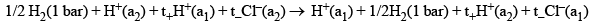Cancelling the comon term, we get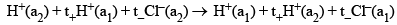which on rearranging gives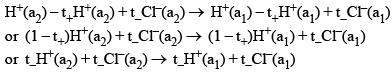Thus, the net cell reaction is to transfer t- mole of HCl from the solution of activity ato that of activity
a1.

Free-energy of cell Reaction

The total free energy change of the net cell reaction is

=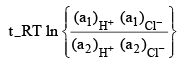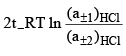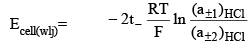Cell without liquid Junction Potential
Electrode reaction at anode 1/2 H2(1 bar) →  H+(a1) + e-
Electrode reaction at cathode H+(a2) + e- →  1/2H2(1 bar)
The net cell reaction is H+(a2)  →  H+(a1)
and the cell potential is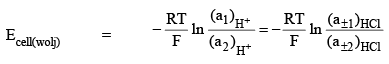where aH+ has been replaced by the mean ionic activity a±.

Expression of liquid junction potential

Elj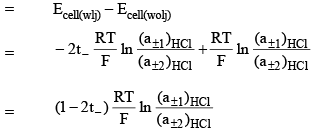Since t+ + t = 1, equation above may be written as

Elj   =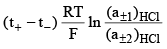Comparing Eqs we get
Ecell(wlj) = 2t–Ecell(wolj)

If the cell without liquid junction is to function s pontaneously, we must have
(a±2)HCl > (a±1)HCl

Comment on liquid junction potential

In a cell with (a±2)HCl > (a±1)HCl, we will have
Elj positive            if t > t

Elj negative           if t < t
and Elj zero          if t = t+

From equation above, we get

Ecell(wlj) > Ecell(wolj)              if t– > t
Ecell(wlj) < Ecell(wolj)            if t– < t+
and Ecell(wlj) = Ecell(wolj)      if t– = t+

CELL IN WHICH ELECTRODES ARE REVERSIBLE WITH RESPECT TO ANIONS

A typical Example Consider the cell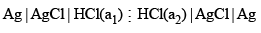Working of the cell

(i)  Electrode reaction at anode Ag(s) + Cl(a1) →  AgCl(s) + e
(ii)  Electrode reaction at cathode AgCl(s) + e→  Ag(s) + Cl-(a2)
(iii)  Migration of H+ ions t+ H+(a1)  →  t+ H+ (a2)
(iv)  Migration of Cl- ions t-Cl-(a2)  →  t-Cl(a1)

The net effect is obtained by adding the above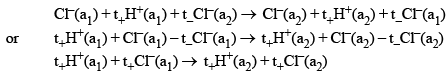Thus, the net cell reaction is to transfer t+ mole of HCl from the solution of activity a1 to that of activity
a2.
The free energy change of the net cell reaction is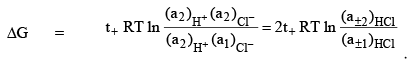Hence Ecell(wlj) =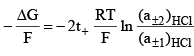Cell without liquid junction potential

Cl(a1)  →  Cl-(a2)

The cell potential would be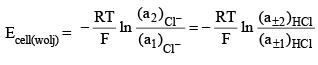where aCl- has been replaced by the mean ionic activity a±.

Expression of liquid Junction potential Now since

Eij = Ecell(wlj) – Ecell(wolj)  we get

Elj =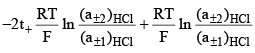or Elj =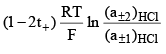or Elj =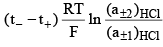Ecell(wlj) = 2t+ Ecell(wolj)

If the cell without liquid junction is to function spontaneously, we must have

(a±1)HCl > (a±2)HCl

Comment on liquid junction potential

In general, the sign and magnitude of the liquid function potential depends on the transference numbers of involved cations and anions. In a cell with
(a±1)HCl > (a±2)HCl,
we have Elj
positive                   if t+ > t Elj
negative                  if t+ < t–
and Elj zero             if t+ = t

From above equation we get
Ecell(wlj) > Ecell(wolj)                 if t+ > t
Ecell(wlj) < Ecell(wolj)                 if t+ < t
and Ecell(wlj) = Ecell(wolj)          if t+ = t–

Generalization of Results

Ecell(wlj) =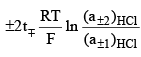Ecell(wlj) =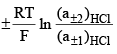Ecell(wlj) =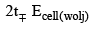Elj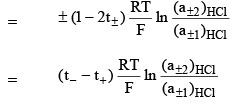Offer running on EduRev: Apply code STAYHOME200 to get INR 200 off on our premium plan EduRev Infinity!

## Physical Chemistry

84 videos|106 docs|31 tests

,

,

,

,

,

,

,

,

,

,

,

,

,

,

,

,

,

,

,

,

,

;